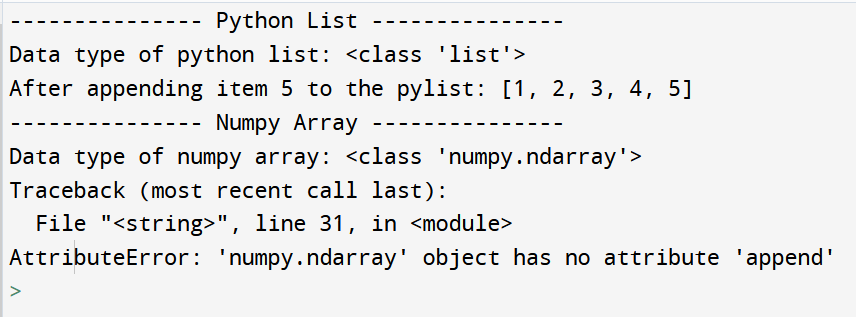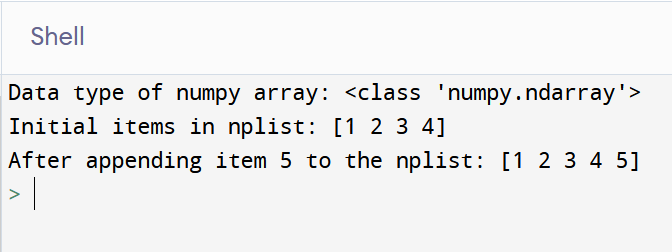Open In App

# How to Fix: ‘numpy.ndarray’ object has no attribute ‘append’

NumPy is a library for the Python programming language, adding support for large, multi-dimensional arrays and matrices, along with a large collection of high-level mathematical functions to operate on these arrays. If you are into analytics, you might have come across this library in python. In the beginning, some things might confuse a programmer when switching from python traditional lists to NumPy arrays. One such error that we might come across is “AttributeError: ‘numpy.ndarray’ object has no attribute ‘append’“.  In this article, let us look at why do we see this error and how to fix it.

When adding an item to a python list, we make use of the list’s append method. The syntax is pretty simple and when we try to replicate the same on a NumPy array we get the above-mentioned error. Let us look through it with an example.

Example: Code that depicts the error

## Python

 `# Append method on python lists`` ` `import` `numpy``print``(``"-"``*``15``, ``"Python List"``, ``"-"``*``15``)`` ` `# Create a python list``pylist ``=` `[``1``, ``2``, ``3``, ``4``]`` ` `# View the data type of the list object``print``(``"Data type of python list:"``, ``type``(pylist))`` ` `# Add (append) an item to the python list``pylist.append(``5``)`` ` `# View the items in the list``print``(``"After appending item 5 to the pylist:"``, pylist)`` ` `print``(``"-"``*``15``, ``"Numpy Array"``, ``"-"``*``15``)`` ` `# Append method on numpy arrays`` ` `# Import the numpy library`` ` `# Create a numpy array``nplist ``=` `numpy.array([``1``, ``2``, ``3``, ``4``])`` ` `# View the data type of the numpy array``print``(``"Data type of numpy array:"``, ``type``(nplist))`` ` `# Add (append) an item to the numpy array``nplist.append(``5``)`

Output:Numpy Append Error

In the above output, we can see that the python list has a data type of list. When we perform the append operation, the item i.e., 5 gets appended to the end of the list `pylist`. While we try the same method for the NumPy array, it fails and throws an error “AttributeError: ‘numpy.ndarray’ object has no attribute ‘append’“. The output is pretty explanatory, the NumPy array has a type of numpy.ndarray which does not have any append() method.

Now, we know that the append is not supported by NumPy arrays then how do we use it? It is actually a method of NumPy and not its array, let us understand it through the example given below, where we actually perform the append operation on a numpy list.

Syntax:

numpy.append(arr, values, axis=None)

Parameters:

• arr: numpy array: The array to which the values are appended to as a copy of it.
• values: numpy array or value: These values are appended to a copy of arr. It must be of the correct shape (the same shape as arr, excluding axis). If axis is not specified, values can be any shape and will be flattened before use.
• axis: int, optional: The axis along which values are appended. If axis is not given, both arr and values are flattened before use.

Example: Fixed code

## Python

 `# Append method on numpy arrays`` ` `# Import the numpy library``import` `numpy`` ` `# Create a numpy array``nplist ``=` `numpy.array([``1``, ``2``, ``3``, ``4``])`` ` `# View the data type of the numpy array``print``(``"Data type of numpy array:"``, ``type``(nplist))`` ` `# View the items in the numpy array``print``(``"Initial items in nplist:"``, nplist)`` ` `# Add (append) an item to the numpy array``nplist ``=` `numpy.append(nplist, ``5``)`` ` `# View the items in the numpy array``print``(``"After appending item 5 to the nplist:"``, nplist)`

Output:Numpy Append Output

As in the output, we can see that initially, the NumPy array had 4 items (1, 2, 3, 4). After appending 5 to the list, it is reflected in the NumPy array. This is so because here the append function is used on NumPy and not on NumPy array object (numpy.ndarray).